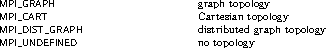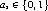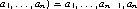## 184. Topology Inquiry FunctionsUp: Topology Constructors Next: Cartesian Shift Coordinates Previous: Distributed Graph Constructor

If a topology has been defined with one of the above functions, then the topology information can be looked up using inquiry functions. They all are local calls.

 MPI_TOPO_TEST(comm, status) IN comm communicator (handle) OUT status topology type of communicator comm (state)

int MPI_Topo_test(MPI_Comm comm, int *status)

MPI_Topo_test(comm, status, ierror)
TYPE(MPI_Comm), INTENT(IN) :: comm
INTEGER, INTENT(OUT) :: status
INTEGER, OPTIONAL, INTENT(OUT) :: ierror
MPI_TOPO_TEST(COMM, STATUS, IERROR)
INTEGER COMM, STATUS, IERROR

The function MPI_TOPO_TEST returns the type of topology that is assigned to a communicator.

The output value status is one of the following:MPI_GRAPHDIMS_GET(comm, nnodes, nedges) IN comm communicator for group with graph structure (handle) OUT nnodes number of nodes in graph (integer) (same as number of processes in the group) OUT nedges number of edges in graph (integer)

int MPI_Graphdims_get(MPI_Comm comm, int *nnodes, int *nedges)

MPI_Graphdims_get(comm, nnodes, nedges, ierror)
TYPE(MPI_Comm), INTENT(IN) :: comm
INTEGER, INTENT(OUT) :: nnodes, nedges
INTEGER, OPTIONAL, INTENT(OUT) :: ierror
MPI_GRAPHDIMS_GET(COMM, NNODES, NEDGES, IERROR)
INTEGER COMM, NNODES, NEDGES, IERROR

Functions MPI_GRAPHDIMS_GET and MPI_GRAPH_GET retrieve the graph-topology information that was associated with a communicator by MPI_GRAPH_CREATE.

The information provided by MPI_GRAPHDIMS_GET can be used to dimension the vectors index and edges correctly for the following call to MPI_GRAPH_GET.

 MPI_GRAPH_GET(comm, maxindex, maxedges, index, edges) IN comm communicator with graph structure (handle) IN maxindex length of vector index in the calling program (integer) IN maxedges length of vector edges in the calling program (integer) OUT index array of integers containing the graph structure (for details see the definition of MPI_GRAPH_CREATE) OUT edges array of integers containing the graph structure

int MPI_Graph_get(MPI_Comm comm, int maxindex, int maxedges, int index[], int edges[])

MPI_Graph_get(comm, maxindex, maxedges, index, edges, ierror)
TYPE(MPI_Comm), INTENT(IN) :: comm
INTEGER, INTENT(IN) :: maxindex, maxedges
INTEGER, INTENT(OUT) :: index(maxindex), edges(maxedges)
INTEGER, OPTIONAL, INTENT(OUT) :: ierror
MPI_GRAPH_GET(COMM, MAXINDEX, MAXEDGES, INDEX, EDGES, IERROR)
INTEGER COMM, MAXINDEX, MAXEDGES, INDEX(*), EDGES(*), IERROR

 MPI_CARTDIM_GET(comm, ndims) IN comm communicator with Cartesian structure (handle) OUT ndims number of dimensions of the Cartesian structure (integer)

int MPI_Cartdim_get(MPI_Comm comm, int *ndims)

MPI_Cartdim_get(comm, ndims, ierror)
TYPE(MPI_Comm), INTENT(IN) :: comm
INTEGER, INTENT(OUT) :: ndims
INTEGER, OPTIONAL, INTENT(OUT) :: ierror
MPI_CARTDIM_GET(COMM, NDIMS, IERROR)
INTEGER COMM, NDIMS, IERROR

The functions MPI_CARTDIM_GET and MPI_CART_GET return the Cartesian topology information that was associated with a communicator by MPI_CART_CREATE. If comm is associated with a zero-dimensional Cartesian topology, MPI_CARTDIM_GET returns ndims=0 and MPI_CART_GET will keep all output arguments unchanged.

 MPI_CART_GET(comm, maxdims, dims, periods, coords) IN comm communicator with Cartesian structure (handle) IN maxdims length of vectors dims, periods, and coords in the calling program (integer) OUT dims number of processes for each Cartesian dimension (array of integer) OUT periods periodicity ( true/ false) for each Cartesian dimension (array of logical) OUT coords coordinates of calling process in Cartesian structure (array of integer)

int MPI_Cart_get(MPI_Comm comm, int maxdims, int dims[], int periods[], int coords[])

MPI_Cart_get(comm, maxdims, dims, periods, coords, ierror)
TYPE(MPI_Comm), INTENT(IN) :: comm
INTEGER, INTENT(IN) :: maxdims
INTEGER, INTENT(OUT) :: dims(maxdims), coords(maxdims)
LOGICAL, INTENT(OUT) :: periods(maxdims)
INTEGER, OPTIONAL, INTENT(OUT) :: ierror
MPI_CART_GET(COMM, MAXDIMS, DIMS, PERIODS, COORDS, IERROR)
INTEGER COMM, MAXDIMS, DIMS(*), COORDS(*), IERROR
LOGICAL PERIODS(*)

 MPI_CART_RANK(comm, coords, rank) IN comm communicator with Cartesian structure (handle) IN coords integer array (of size ndims) specifying the Cartesian coordinates of a process OUT rank rank of specified process (integer)

int MPI_Cart_rank(MPI_Comm comm, const int coords[], int *rank)

MPI_Cart_rank(comm, coords, rank, ierror)
TYPE(MPI_Comm), INTENT(IN) :: comm
INTEGER, INTENT(IN) :: coords(*)
INTEGER, INTENT(OUT) :: rank
INTEGER, OPTIONAL, INTENT(OUT) :: ierror
MPI_CART_RANK(COMM, COORDS, RANK, IERROR)
INTEGER COMM, COORDS(*), RANK, IERROR

For a process group with Cartesian structure, the function MPI_CART_RANK translates the logical process coordinates to process ranks as they are used by the point-to-point routines.

For dimension i with periods(i) = true, if the coordinate, coords(i), is out of range, that is, coords(i) < 0 or coords(i)dims(i), it is shifted back to the interval 0coords(i) < dims(i) automatically. Out-of-range coordinates are erroneous for non-periodic dimensions.

If comm is associated with a zero-dimensional Cartesian topology, coords is not significant and 0 is returned in rank.

 MPI_CART_COORDS(comm, rank, maxdims, coords) IN comm communicator with Cartesian structure (handle) IN rank rank of a process within group of comm (integer) IN maxdims length of vector coords in the calling program (integer) OUT coords integer array (of size ndims) containing the Cartesian coordinates of specified process (array of integers)

int MPI_Cart_coords(MPI_Comm comm, int rank, int maxdims, int coords[])

MPI_Cart_coords(comm, rank, maxdims, coords, ierror)
TYPE(MPI_Comm), INTENT(IN) :: comm
INTEGER, INTENT(IN) :: rank, maxdims
INTEGER, INTENT(OUT) :: coords(maxdims)
INTEGER, OPTIONAL, INTENT(OUT) :: ierror
MPI_CART_COORDS(COMM, RANK, MAXDIMS, COORDS, IERROR)
INTEGER COMM, RANK, MAXDIMS, COORDS(*), IERROR

The inverse mapping, rank-to-coordinates translation is provided by MPI_CART_COORDS. If comm is associated with a zero-dimensional Cartesian topology, coords will be unchanged.

 MPI_GRAPH_NEIGHBORS_COUNT(comm, rank, nneighbors) IN comm communicator with graph topology (handle) IN rank rank of process in group of comm (integer) OUT nneighbors number of neighbors of specified process (integer)

int MPI_Graph_neighbors_count(MPI_Comm comm, int rank, int *nneighbors)

MPI_Graph_neighbors_count(comm, rank, nneighbors, ierror)
TYPE(MPI_Comm), INTENT(IN) :: comm
INTEGER, INTENT(IN) :: rank
INTEGER, INTENT(OUT) :: nneighbors
INTEGER, OPTIONAL, INTENT(OUT) :: ierror
MPI_GRAPH_NEIGHBORS_COUNT(COMM, RANK, NNEIGHBORS, IERROR)
INTEGER COMM, RANK, NNEIGHBORS, IERROR

 MPI_GRAPH_NEIGHBORS(comm, rank, maxneighbors, neighbors) IN comm communicator with graph topology (handle) IN rank rank of process in group of comm (integer) IN maxneighbors size of array neighbors (integer) OUT neighbors ranks of processes that are neighbors to specified process (array of integer)

int MPI_Graph_neighbors(MPI_Comm comm, int rank, int maxneighbors, int neighbors[])

MPI_Graph_neighbors(comm, rank, maxneighbors, neighbors, ierror)
TYPE(MPI_Comm), INTENT(IN) :: comm
INTEGER, INTENT(IN) :: rank, maxneighbors
INTEGER, INTENT(OUT) :: neighbors(maxneighbors)
INTEGER, OPTIONAL, INTENT(OUT) :: ierror
MPI_GRAPH_NEIGHBORS(COMM, RANK, MAXNEIGHBORS, NEIGHBORS, IERROR)
INTEGER COMM, RANK, MAXNEIGHBORS, NEIGHBORS(*), IERROR

MPI_GRAPH_NEIGHBORS_COUNT and MPI_GRAPH_NEIGHBORS provide adjacency information for a graph topology. The returned count and array of neighbors for the queried rank will both include all neighbors and reflect the same edge ordering as was specified by the original call to MPI_GRAPH_CREATE. Specifically, MPI_GRAPH_NEIGHBORS_COUNT and MPI_GRAPH_NEIGHBORS will return values based on the original index and edges array passed to MPI_GRAPH_CREATE (for the purpose of this example, we assume that index[-1] is zero):

• The number of neighbors ( nneighbors) returned from MPI_GRAPH_NEIGHBORS_COUNT will be ( index[rank] - index[rank-1]).
• The neighbors array returned from MPI_GRAPH_NEIGHBORS will be edges[index[rank-1]] through edges[index[rank]-1].

Example Assume there are four processes 0, 1, 2, 3 with the following adjacency matrix (note that some neighbors are listed multiple times):

 process neighbors 0 1, 1, 3 1 0, 0 2 3 3 0, 2, 2

Thus, the input arguments to MPI_GRAPH_CREATE are:

 nnodes = 4 index = 3, 5, 6, 9 edges = 1, 1, 3, 0, 0, 3, 0, 2, 2

Therefore, calling MPI_GRAPH_NEIGHBORS_COUNT and MPI_GRAPH_NEIGHBORS for each of the 4 processes will return:

 Input rank Count Neighbors 0 3 1, 1, 3 1 2 0, 0 2 1 3 3 3 0, 2, 2

Example Suppose that comm is a communicator with a shuffle-exchange topology. The group has 2n members. Each process is labeled by a1 , ..., an with, and has three neighbors: exchange((), shuffle(a1 , ..., an )= a2 , ..., an, a1, and unshuffle(a1 , ..., an ) = an , a1 , ... , an-1. The graph adjacency list is illustrated below for n=3.

 node exchange shuffle unshuffle neighbors(1) neighbors(2) neighbors(3) 0 (000) 1 0 0 1 (001) 0 2 4 2 (010) 3 4 1 3 (011) 2 6 5 4 (100) 5 1 2 5 (101) 4 3 6 6 (110) 7 5 3 7 (111) 6 7 7

Suppose that the communicator comm has this topology associated with it. The following code fragment cycles through the three types of neighbors and performs an appropriate permutation for each.

```!  assume: each process has stored a real number A.
!  extract neighborhood information
CALL MPI_COMM_RANK(comm, myrank, ierr)
CALL MPI_GRAPH_NEIGHBORS(comm, myrank, 3, neighbors, ierr)
!  perform exchange permutation
CALL MPI_SENDRECV_REPLACE(A, 1, MPI_REAL, neighbors(1), 0, &
neighbors(1), 0, comm, status, ierr)
!  perform shuffle permutation
CALL MPI_SENDRECV_REPLACE(A, 1, MPI_REAL, neighbors(2), 0, &
neighbors(3), 0, comm, status, ierr)
!  perform unshuffle permutation
CALL MPI_SENDRECV_REPLACE(A, 1, MPI_REAL, neighbors(3), 0, &
neighbors(2), 0, comm, status, ierr)
```

MPI_DIST_GRAPH_NEIGHBORS_COUNT and MPI_DIST_GRAPH_NEIGHBORS provide adjacency information for a distributed graph topology.

 MPI_DIST_GRAPH_NEIGHBORS_COUNT(comm, indegree, outdegree, weighted) IN comm communicator with distributed graph topology (handle) OUT indegree number of edges into this process (non-negative integer) OUT outdegree number of edges out of this process (non-negative integer) OUT weighted false if MPI_UNWEIGHTED was supplied during creation, true otherwise (logical)

int MPI_Dist_graph_neighbors_count(MPI_Comm comm, int *indegree, int *outdegree, int *weighted)

MPI_Dist_graph_neighbors_count(comm, indegree, outdegree, weighted, ierror)
TYPE(MPI_Comm), INTENT(IN) :: comm
INTEGER, INTENT(OUT) :: indegree, outdegree
LOGICAL, INTENT(OUT) :: weighted
INTEGER, OPTIONAL, INTENT(OUT) :: ierror
MPI_DIST_GRAPH_NEIGHBORS_COUNT(COMM, INDEGREE, OUTDEGREE, WEIGHTED, IERROR)
INTEGER COMM, INDEGREE, OUTDEGREE, IERROR
LOGICAL WEIGHTED

 MPI_DIST_GRAPH_NEIGHBORS(comm, maxindegree, sources, sourceweights, maxoutdegree, destinations, destweights) IN comm communicator with distributed graph topology (handle) IN maxindegree size of sources and sourceweights arrays (non-negative integer) OUT sources processes for which the calling process is a destination (array of non-negative integers) OUT sourceweights weights of the edges into the calling process (array of non-negative integers) IN maxoutdegree size of destinations and destweights arrays (non-negative integer) OUT destinations processes for which the calling process is a source (array of non-negative integers) OUT destweights weights of the edges out of the calling process (array of non-negative integers)

int MPI_Dist_graph_neighbors(MPI_Comm comm, int maxindegree, int sources[], int sourceweights[], int maxoutdegree, int destinations[], int destweights[])

MPI_Dist_graph_neighbors(comm, maxindegree, sources, sourceweights, maxoutdegree, destinations, destweights, ierror)
TYPE(MPI_Comm), INTENT(IN) :: comm
INTEGER, INTENT(IN) :: maxindegree, maxoutdegree
INTEGER, INTENT(OUT) :: sources(maxindegree),
destinations(maxoutdegree)
INTEGER :: sourceweights(*), destweights(*)
INTEGER, OPTIONAL, INTENT(OUT) :: ierror
MPI_DIST_GRAPH_NEIGHBORS(COMM, MAXINDEGREE, SOURCES, SOURCEWEIGHTS, MAXOUTDEGREE, DESTINATIONS, DESTWEIGHTS, IERROR)
INTEGER COMM, MAXINDEGREE, SOURCES(*), SOURCEWEIGHTS(*), MAXOUTDEGREE,
DESTINATIONS(*), DESTWEIGHTS(*), IERROR

These calls are local. The number of edges into and out of the process returned by MPI_DIST_GRAPH_NEIGHBORS_COUNT are the total number of such edges given in the call to MPI_DIST_GRAPH_CREATE_ADJACENT or MPI_DIST_GRAPH_CREATE (potentially by processes other than the calling process in the case of MPI_DIST_GRAPH_CREATE). Multiply defined edges are all counted and returned by MPI_DIST_GRAPH_NEIGHBORS in some order. If MPI_UNWEIGHTED is supplied for sourceweights or destweights or both, or if MPI_UNWEIGHTED was supplied during the construction of the graph then no weight information is returned in that array or those arrays. If the communicator was created with MPI_DIST_GRAPH_CREATE_ADJACENT then for each rank in comm, the order of the values in sources and destinations is identical to the input that was used by the process with the same rank in comm_old in the creation call. If the communicator was created with MPI_DIST_GRAPH_CREATE then the only requirement on the order of values in sources and destinations is that two calls to the routine with same input argument comm will return the same sequence of edges. If maxindegree or maxoutdegree is smaller than the numbers returned by MPI_DIST_GRAPH_NEIGHBOR_COUNT, then only the first part of the full list is returned.